# Python | Pandas DataFrame.tz_localize

• Last Updated : 30 May, 2022

Pandas DataFrame is a two-dimensional size-mutable, potentially heterogeneous tabular data structure with labeled axes (rows and columns). Arithmetic operations align on both row and column labels. It can be thought of as a dict-like container for Series objects. This is the primary data structure of the Pandas. Pandas DataFrame.tz_localize() function localize tz-naive index of a Series or DataFrame to target time zone. This operation localizes the Index.

Syntax: DataFrame.tz_localize(tz, axis=0, level=None, copy=True, ambiguous=’raise’, nonexistent=’raise’) Parameter : tz : string or pytz.timezone object axis : the axis to localize level : If axis is a MultiIndex, localize a specific level. Otherwise must be None copy : Also make a copy of the underlying data ambiguous : When clocks moved backward due to DST, ambiguous times may arise nonexistent : A nonexistent time does not exist in a particular timezone where clocks moved forward due to DST Returns : Same type as the input.

Example #1: Use DataFrame.tz_localize() function to localize the given tz-naive index of the dataframe to the target timezone.

## Python3

 `# importing pandas as pd` `import` `pandas as pd`   `# Creating the DataFrame` `df ``=` `pd.DataFrame({``'Weight'``:[``45``, ``88``, ``56``, ``15``, ``71``],` `                   ``'Name'``:[``'Sam'``, ``'Andrea'``, ``'Alex'``, ``'Robin'``, ``'Kia'``],` `                   ``'Age'``:[``14``, ``25``, ``55``, ``8``, ``21``]})`   `# Create the index` `index_ ``=` `pd.date_range(``'2010-10-09 08:45'``, periods ``=` `5``, freq ``=``'H'``, tz ``=` `'US / Central'``)`   `# Set the index` `df.index ``=` `index_`   `# Print the DataFrame` `print``(df)`

Output :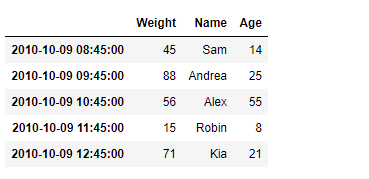Now we will use DataFrame.tz_localize() function to localize the given tz-naive index of the dataframe to the ‘Europe/Berlin’ timezone.

## Python3

 `# Let's find out the current timezone` `# of the given dataframe` `print``(df.index)`   `# Let's localize the timezone of the` `# dataframe index to 'Europe / Berlin'` `df ``=` `df ``=` `df.tz_localize(tz ``=` `'Europe / Berlin'``)`   `# Let's find out the current timezone` `# of the given dataframe` `print``(df.index) `

Output :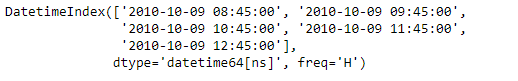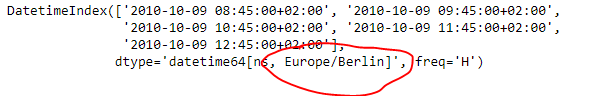As we can see in the output, the DataFrame.tz_localize() function has successfully localized the tz-naive index of the given dataframe to the target timezone.   Example #2 : Use DataFrame.tz_localize() function to localize the tz-naive Index of the given dataframe. The Index of the given dataframe is a MultiIndex.

## Python3

 `# importing pandas as pd` `import` `pandas as pd`   `# Creating the DataFrame` `df ``=` `pd.DataFrame({``'Weight'``:[``45``, ``88``, ``56``, ``15``, ``71``],` `                   ``'Name'``:[``'Sam'``, ``'Andrea'``, ``'Alex'``, ``'Robin'``, ``'Kia'``],` `                   ``'Age'``:[``14``, ``25``, ``55``, ``8``, ``21``]})`   `# Create the MultiIndex` `index_ ``=` `pd.MultiIndex.from_product([[``'Date'``], pd.date_range(``'2010-10-09 08:45'``, periods ``=` `5``, freq ``=``'H'``)],` `           ``names ``=``[``'Level 1'``, ``'Level 2'``])`   `# Set the index` `df.index ``=` `index_`   `# Print the DataFrame` `print``(df)`

Output :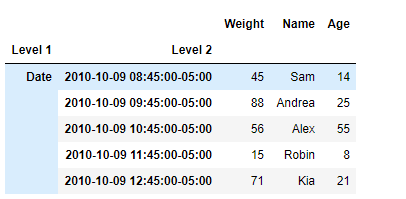Now we will use DataFrame.tz_localize() function to localize the tz-naive index of the given dataframe to ‘US/Central’.

## Python3

 `# Let's find out the current timezone` `# of the Level 1 of the given dataframe` `print``(df.index[``1``])`   `# Let's localize the timezone of the` `# level 1 of the dataframe to 'US / Central'` `df ``=` `df.tz_localize(tz ``=` `'US / Central'``, level ``=` `1``)`   `# Let's find out the current timezone` `# of the level 1 of the given dataframe` `print``(df.index[``1``]) `

Output :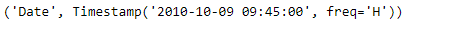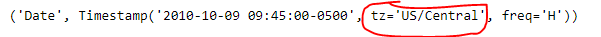As we can see in the output, the DataFrame.tz_localize() function has successfully localized the tz-naive index of the given dataframe to ‘US/Central’.

My Personal Notes arrow_drop_up
Recommended Articles
Page :